# HashMap 底层实现原理分析

`HashMap``Map` 的一个实现类，它代表的是一种键值对的数据存储形式。

`HashMap`最多只允许一条记录的键为`null`，允许多条记录的值为`null`。不保证有序 (比如插入的顺序)、也不保证序不随时间变化。

`jdk 8` 之前，其内部是由 数组 + 链表 来实现的，而 `jdk 8` 对于链表长度超过 8 的链表将转储为 红黑树。

`HashMap`非线程安全，即任一时刻可以有多个线程同时写`HashMap`，可能会导致数据的不一致。如果需要满足线程安全，可以用 `Collections``synchronizedMap`方法使`HashMap`具有线程安全的能力，或者使用`ConcurrentHashMap`

`HashMap`是 数组 + 链表 + 红黑树（JDK1.8 增加了红黑树部分）实现的。JDK 1.8 之所以添加红黑树是因为一旦链表过长，会严重影响 HashMap 的性能，而红黑树具有快速增删改查的特点，这样就可以有效的解决链表过长时操作比较慢的问题。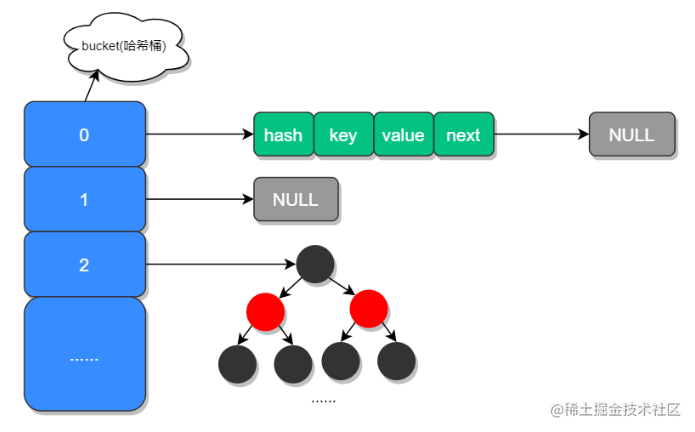## 存储结构

``````static class Node<K,V> implements Map.Entry<K,V> {
final int hash;
final K key;
V value;
Node<K,V> next;

Node(int hash, K key, V value, Node<K,V> next) {
this.hash = hash;
this.key = key;
this.value = value;
this.next = next;
}

public final K getKey()        { return key; }
public final V getValue()      { return value; }
public final String toString() { return key + "=" + value; }

public final int hashCode() {
return Objects.hashCode(key) ^ Objects.hashCode(value);
}

public final V setValue(V newValue) {
V oldValue = value;
value = newValue;
return oldValue;
}

public final boolean equals(Object o) {
if (o == this)
return true;
if (o instanceof Map.Entry) {
Map.Entry<?,?> e = (Map.Entry<?,?>)o;
if (Objects.equals(key, e.getKey()) &&
Objects.equals(value, e.getValue()))
return true;
}
return false;
}
}

`Node``HashMap`的一个内部类，实现了 Map.Entry 接口，本质上就是一个映射 (键值对)。

`HashMap`类中有一个非常重要的字段，就是 `Node[] table`，即哈希桶数组。如果哈希桶数组很大，即使较差的`Hash`算法也会比较分散，如果哈希桶数组数组很小，即使好的`Hash`算法也会出现较多碰撞。

• HashMap 中的hash算法如何实现？
• JDK1.8HashMap扩容时做了哪些优化？
• 加载因子为什么是0.75？
• 当有哈希冲突时，HashMap是如何查找并确认元素的？HashMap源码中有哪些重要的方法？
• HashMap 源码中有哪些重要的方法？

## 成员变量

``````// HashMap 初始化长度
static final int DEFAULT_INITIAL_CAPACITY = 1 << 4;
// HashMap 最大长度
static final int MAXIMUM_CAPACITY = 1 << 30;
// 默认的加载因子 （扩容因子）
static final float DEFAULT_LOAD_FACTOR = 0.75f;
// 当链表长度大于此值且容量大于64时
static final int TREEIFY_THRESHOLD = 8;
// 转换链表的临界值，当元素小于此值时，将红黑树转换为链表结构
static final int UNTREEIFY_THRESHOLD = 6;
// 最小数容量
static final int MIN_TREEIFY_CAPACITY = 64;

transient Node<K,V>[] table;

transient Set<Map.Entry<K,V>> entrySet;

transient int size;

transient int modCount;

int threshold;

`Capacity`就是`buckets`的数目，`Load factor`就是`buckets`填满程度的最大比例。如果对迭代性能要求很高的话不要把`capacity`设置过大，也不要把`load factor`设置过小。当`bucket`填充的数目（即 hashmap 中元素的个数）大于`capacity*load factor`时就需要调整 buckets 的数目为当前的 2 倍

## HashMap 中的hash算法如何实现？

``````static final int hash(Object key) {
int h;
return (key == null) ? 0 : (h = key.hashCode()) ^ (h >>> 16);
}

static int indexFor(int h, int length) {
return h & (length-1);
}

indexFor 是 jdk1.7 的源码，jdk1.8 没有这个方法但是 jdk1.8 也是通过取模运算来计算的

1. 取 key 的 hashCode 值
2. 高位运算
3. 取模运算

`JDK1.8`的实现中，优化了高位运算的算法，通过`hashCode()`的高 16 位异或低 16 位实现的：(h = k.hashCode()) ^ (h >>> 16)，主要是从速度、功效、质量来考虑的，这么做可以 Node 数组 table 的 length 比较小的时候，也能保证考虑到高低 Bit 都参与到 Hash 的计算中，同时不会有太大的开销。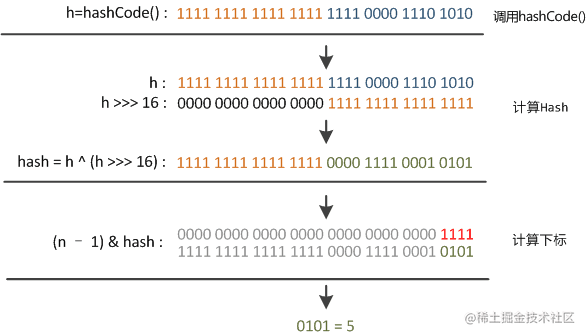## JDK1.8HashMap扩容时做了哪些优化？

`put`时，如果发现目前的`bucket`占用程度已经超过了`Load Factor`所希望的比例，那么就会发生`resize`。在`resize`的过程，简单的说就是把`bucket`扩充为 2 倍，之后重新计算`index`，把节点再放到新的`bucket`中。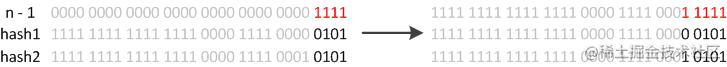``````final Node<K,V>[] resize() {
// 扩容前的数组
Node<K,V>[] oldTab = table;
// 扩容前的数组大小和阈值
int oldCap = (oldTab == null) ? 0 : oldTab.length;
int oldThr = threshold;
// 预定义新数组的大小和阈值
int newCap, newThr = 0;
if (oldCap > 0) {
// 超过最大值就不再扩容了
if (oldCap >= MAXIMUM_CAPACITY) {
threshold = Integer.MAX_VALUE;
return oldTab;
}
// 扩大容量为当前容量的两倍，但不能超过 MAXIMUM_CAPACITY
else if ((newCap = oldCap << 1) < MAXIMUM_CAPACITY &&
oldCap >= DEFAULT_INITIAL_CAPACITY)
newThr = oldThr << 1;
}
// 当前数组没有数据，使用初始化的值
else if (oldThr > 0)
newCap = oldThr;
else {
// 如果初始化的值为 0， 则使用默认的初始化容量
newCap = DEFAULT_INITIAL_CAPACITY;
}

if (newThr == 0) {
float ft = (float)newCap * loadFactor;
newThr = (newCap < MAXIMUM_CAPACITY && ft < (float)MAXIMUM_CAPACITY ?
(int)ft : Integer.MAX_VALUE);
}
threshold = newThr;
@SuppressWarnings({"rawtypes","unchecked"})
Node<K,V>[] newTab = (Node<K,V>[])new Node[newCap];

// 开始扩容，将新的容量赋值给 table
table = newTab;
// 原数据不为空，将原始的容量复制到新 table 中
if (oldTab != null) {
// 根据容量循环数组，复制非空元素到新 table
for (int j = 0; j < oldCap; ++j) {
Node<K,V> e;
if ((e = oldTab[j]) != null) {
oldTab[j] = null;
// 如果链表只有一个，则进行直接赋值
if (e.next == null)
newTab[e.hash & (newCap - 1)] = e;
else if (e instanceof TreeNode)
// 红黑树相关的操作
((TreeNode<K,V>)e).split(this, newTab, j, oldCap);
else {
// 链表复制,JDK 1.8 扩容优化部分
Node<K,V> loHead = null, loTail = null;
Node<K,V> hiHead = null, hiTail = null;
Node<K,V> next;
do {
next = e.next;
// 原索引
if ((e.hash & oldCap) == 0) {
if (loTail == null)
else
loTail.next = e;
loTail = e;
}
// 原索引 + oldCap
else {
if (hiTail == null)
else
hiTail.next = e;
hiTail = e;
}
} while ((e = next) != null);
// 将原索引放入到哈希桶中
if (loTail != null) {
loTail.next = null;
}
// 将原索引 + oldCap 放到哈希桶中
if (hiTail != null) {
hiTail.next = null;
}
}
}
}
}
return newTab;
}

## 加载因子为什么是0.75？

• 当加载因子设置比较大的时候，扩容的门槛就被提高了，扩容发生的频率比较低，占用的空间会比较小，但此时发生 Hash 冲突的几率就会提升，因此需要更复杂的数据结构来存储元素，这样对元素的操作时间就会增加，运行效率也会因此降低；
• 而当加载因子值比较小的时候，扩容的门槛会比较低，因此会占用更多的空间，此时元素的存储就比较稀疏，发生哈希冲突的可能性就比较小，因此操作性能会比较高。

• 当有哈希冲突时，HashMap是如何查找并确认元素的？
• HashMap 源码中有哪些重要的方法？

## HashMap 中的核心方法

HashMap 源码中三个重要方法：查询、新增和数据扩容。

### 查询

bucket 里的第一个节点，直接命中；

``````public V get(Object key) {
Node<K,V> e;
// 对 key 进行哈希操作
return (e = getNode(hash(key), key)) == null ? null : e.value;
}
final Node<K,V> getNode(int hash, Object key) {
Node<K,V>[] tab; Node<K,V> first, e; int n; K k;
// 非空判断
if ((tab = table) != null && (n = tab.length) > 0 &&
(first = tab[(n - 1) & hash]) != null) {

// 判断第一个元素是否是要查询的元素
if (first.hash == hash &&
((k = first.key) == key || (key != null && key.equals(k))))
return first;
// 一下一个节点非空判断
if ((e = first.next) != null) {
// 如果第一节点是树结构，则使用 getTreeNode 直接获取相应的数据
if (first instanceof TreeNode)
return ((TreeNode<K,V>)first).getTreeNode(hash, key);
// 非树结构循环节点判断
do {
// hash 相等并且 key 相同，则返回此节点
if (e.hash == hash &&
((k = e.key) == key || (key != null && key.equals(k))))
return e;
} while ((e = e.next) != null);
}
}
return null;
}

### 新增

`put` 方法也是`HashMap`中比较重要的方法，因为通过该方法我们可以窥探到 `HashMap` 在内部是如何进行数据存储的，所谓的 数组 + 链表 + 红黑树的存储结构是如何形成的，又是在何种情况下将链表转换成红黑树来优化性能的。

put 方法的大致实现过程如下：

• 对 key 的 hashCode() 做 hash，然后再计算 index;
• 如果没碰撞直接放到 bucket 里；
• 如果碰撞了，以链表的形式存在 buckets 后；
• 如果碰撞导致链表过长 (大于等于 TREEIFY_THRESHOLD)，就把链表转换成红黑树；
• 如果节点已经存在就替换 old value(保证 key 的唯一性)
• 如果 bucket 满了 (超过 load factor*current capacity)，就要 resize。
``````public V put(K key, V value) {
// 对 key 进行哈希操作
return putVal(hash(key), key, value, false, true);
}

final V putVal(int hash, K key, V value, boolean onlyIfAbsent,
boolean evict) {
Node<K,V>[] tab; Node<K,V> p; int n, i;

// 哈希表为空则创建
if ((tab = table) == null || (n = tab.length) == 0)
n = (tab = resize()).length;
// 根据 key 的哈希值计算出要插入的数组索引 i
if ((p = tab[i = (n - 1) & hash]) == null)
// 如果 table[i] = null 则直接插入
tab[i] = newNode(hash, key, value, null);
else {
Node<K,V> e; K k;
// 如果 key 已经存在了，直接覆盖 value
if (p.hash == hash &&
((k = p.key) == key || (key != null && key.equals(k))))
e = p;
// 如果 key 不存在，判断是否为红黑树
else if (p instanceof TreeNode)
// 红黑树直接插入键值对
e = ((TreeNode<K,V>)p).putTreeVal(this, tab, hash, key, value);
else {
// 链表结构，循环准备插入
for (int binCount = 0; ; ++binCount) {
// 下一个元素为空时
if ((e = p.next) == null) {
p.next = newNode(hash, key, value, null);
// 转换为红黑树进行处理
if (binCount >= TREEIFY_THRESHOLD - 1)
treeifyBin(tab, hash);
break;
}
// key 已经存在直接覆盖 value
if (e.hash == hash &&
((k = e.key) == key || (key != null && key.equals(k))))
break;
p = e;
}
}
if (e != null) {
V oldValue = e.value;
if (!onlyIfAbsent || oldValue == null)
e.value = value;
afterNodeAccess(e);
return oldValue;
}
}
++modCount;

// 超过最大容量，扩容
if (++size > threshold)
resize();
afterNodeInsertion(evict);
return null;
}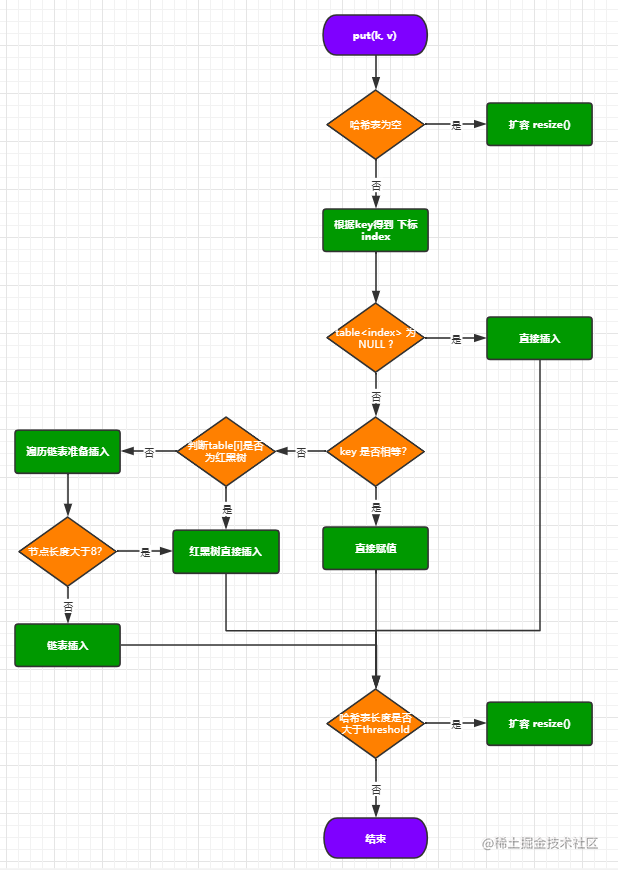## HashMap 死循环分析

``````void transfer(Entry[] newTable, boolean rehash) {
int newCapacity = newTable.length;
for (Entry<K,V> e: table) {
while (null != e) {
Entry<K,V> next = e.next; // 线程一执行到此处
if(rehash) {
e.hash = null == e.key ? 0 : hash(key);
}
int i = indexFor(e.hash, newCapacity);
e.next = newTable[i];
newTable[i] = e;
e = next;
}
}
}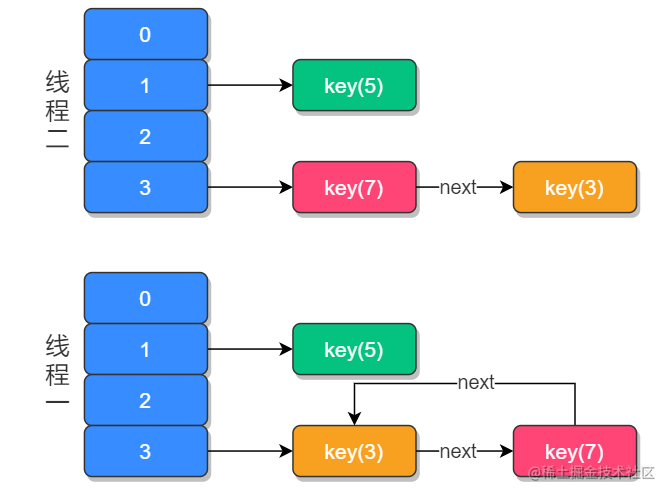## 其他方法

### 构造方法

``````public HashMap(int initialCapacity, float loadFactor) {
if (initialCapacity < 0)
throw new IllegalArgumentException("Illegal initial capacity: " +
initialCapacity);
if (initialCapacity > MAXIMUM_CAPACITY)
initialCapacity = MAXIMUM_CAPACITY;
throw new IllegalArgumentException("Illegal load factor: " +
this.threshold = tableSizeFor(initialCapacity);
}

``````static final int tableSizeFor(int cap) {
int n = cap - 1;
n |= n >>> 1;
n |= n >>> 2;
n |= n >>> 4;
n |= n >>> 8;
n |= n >>> 16;
return (n < 0) ? 1 : (n >= MAXIMUM_CAPACITY) ? MAXIMUM_CAPACITY : n + 1;
}

``````int n = cap - 1

``````n |= n >>> 1;

``````n |= n >>> 2;

``````n |= n >>> 4;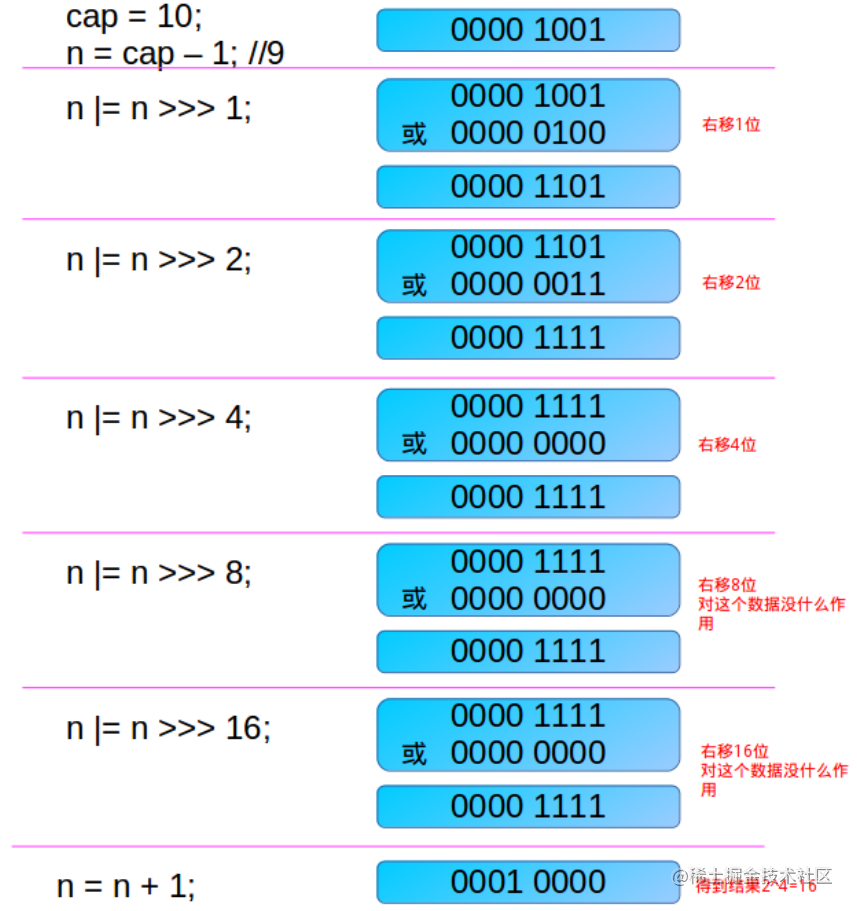HashMap 中还有很多的重载构造函数，但几乎都是基于上述的构造函数的。

``````public HashMap(int initialCapacity) {
}

public HashMap() {
}

``````public HashMap(Map<? extends K, ? extends V> m) {
putMapEntries(m, false);
}

### remove 方法

``````public V remove(Object key) {
Node<K,V> e;
return (e = removeNode(hash(key), key, null, false, true)) == null ?
null : e.value;
}

``````final Node<K,V> removeNode(int hash, Object key, Object value,boolean matchValue, boolean movable) {
Node<K,V>[] tab; Node<K,V> p; int n, index;
if ((tab = table) != null && (n = tab.length) > 0 &&
(p = tab[index = (n - 1) & hash]) != null) {
Node<K,V> node = null, e; K k; V v;
if (p.hash == hash &&
((k = p.key) == key || (key != null && key.equals(k))))
node = p;
else if ((e = p.next) != null) {
if (p instanceof TreeNode)
node = ((TreeNode<K,V>)p).getTreeNode(hash, key);
else {
do {
if (e.hash == hash &&
((k = e.key) == key ||
(key != null && key.equals(k)))) {
node = e;
break;
}
p = e;
} while ((e = e.next) != null);
}
}
if (node != null && (!matchValue || (v = node.value) == value ||(value != null && value.equals(v)))) {
if (node instanceof TreeNode)                                                                     ((TreeNode<K,V>)node).removeTreeNode(this, tab, movable);
else if (node == p)
tab[index] = node.next;
else
p.next = node.next;
++modCount;
--size;
afterNodeRemoval(node);
return node;
}
}
return null;
}

``````if (p.hash == hash &&((k = p.key) == key || (key != null && key.equals(k))))
node = p

``````else if ((e = p.next) != null) {
if (p instanceof TreeNode)
node = ((TreeNode<K,V>)p).getTreeNode(hash, key);
else {
do {
if (e.hash == hash &&((k = e.key) == key ||(key != null && key.equals(k)))) {
node = e;
break;
}
p = e;
} while ((e = e.next) != null);
}
}

``````if (node != null && (!matchValue || (v = node.value) == value ||(value != null && value.equals(v)))) {
if (node instanceof TreeNode)
((TreeNode<K,V>)node).removeTreeNode(this, tab, movable);
else if (node == p)
tab[index] = node.next;
else
p.next = node.next;
++modCount;
--size;
afterNodeRemoval(node);
return node;
}

### keySet

``````transient volatile Set<K>        keySet;

public Set<K> keySet() {
Set<K> ks;
return (ks = keySet) == null ? (keySet = new KeySet()) : ks;
}
final class KeySet extends AbstractSet<K> {
public final int size()                 { return size; }
public final void clear()               { HashMap.this.clear(); }
public final Iterator<K> iterator()     { return new KeyIterator(); }
public final boolean contains(Object o) { return containsKey(o); }
public final boolean remove(Object key) {
return removeNode(hash(key), key, null, false, true) != null;
}
public final Spliterator<K> spliterator() {
return new KeySpliterator<>(HashMap.this, 0, -1, 0, 0);
}
}

HashMap 中定义了一个 keySet 的实例属性，它保存的是整个 HashMap 中所有键的集合。上述所列出的 KeySet 类是 Set 的一个实现类，它负责为我们提供有关 HashMap 中所有对键的操作。